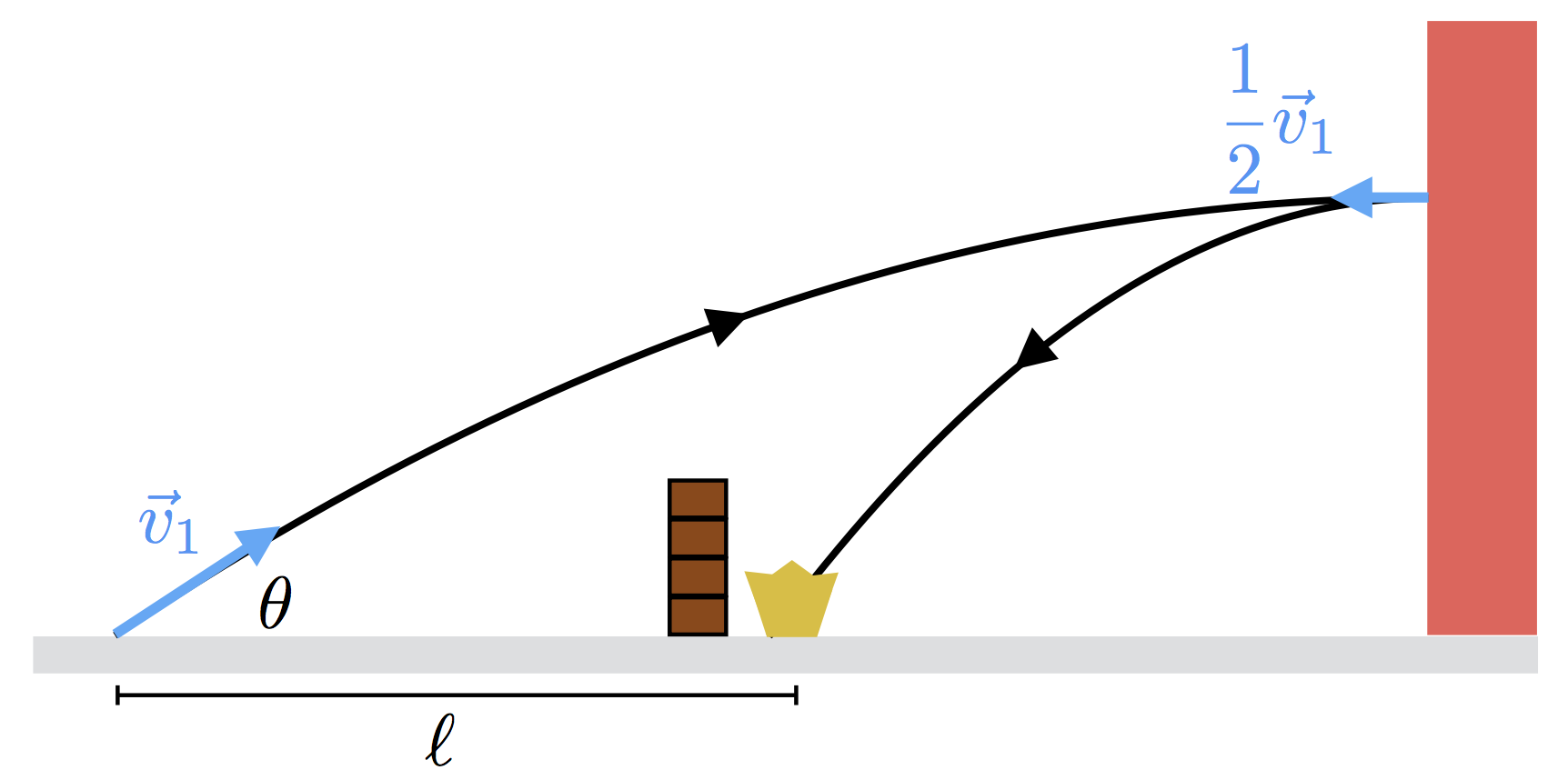# projectors

There's a war on the playground, and you're the charismatic leader of the Greasers. The Socs, your sworn enemies, have fashioned themselves a defensive fort, behind which they're hiding their prized possession, the crown.

The only way to win the war is by making a direct hit on the crown with a tennis ball. Blocked from a direct hit by the fort, the Greasers have to shoot it off the wall behind the fort and bank it into the crown.As shown in the diagram, the Greasers launch a tennis ball with speed $v_1 = \SI[per-mode=symbol]{20}{\meter\per\second}$ at an angle of $\theta=\SI{\frac{\pi}6}{\radian}$ with the horizontal. At the peak of its trajectory, the ball hits the wall and reflects with speed $\frac12 v_1.$

If the ball hits the crown as shown in the diagram, calculate the distance $\ell$ (in $\si{\meter}$) between the launch point and the crown.

Assume that $g = \SI[per-mode=symbol]{9.81}{\meter\per\second\squared}.$

×